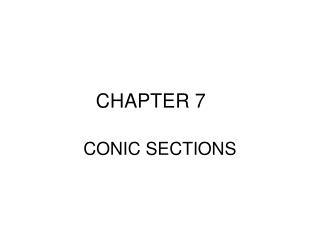DownloadDownload PresentationCHAPTER 7

# CHAPTER 7

Download Presentation## CHAPTER 7

- - - - - - - - - - - - - - - - - - - - - - - - - - - E N D - - - - - - - - - - - - - - - - - - - - - - - - - - -
##### Presentation Transcript

1. CHAPTER 7 CONIC SECTIONS

2. 7.1 THE ELLIPSE • Objectives • Graph ellipses centered at the origin • Write equations of ellipses in standard form • Graph ellipses not centered at the origin • Solve applied problems involving ellipses

3. Definition of an ellipse • All points in a plane the sum of whose distances from 2 fixed points (foci) is constant. • If an ellipse has a center at the origin and the horizontal axis is 2a (distance from center to right end is a) and the vertical axis is 2b (distance from the center to the top is b), the equation of the ellipse is:

4. Graph • Center is at the origin, horizontal axis=6 (left endpt (-3,0), right endpt (3,0), vertical axis = 8 (top endpt (0,4), bottom endpt (0,-4))

5. What is c? • c is the distance from the center to the focal point

6. What is the equation of an ellipse, centered at the origin with a horizontal axis=10 and vertical axis=8?

7. What if the ellipse is not centered at the origin? • If it is centered at any point, (h,k), the ellipse is translated. It is moved right “h” units and up “k” units from the origin. • Consider: • The center is at (2,-3), the distance from the center to the right & left endpoint = 2, the distance to the top & bottom endpoint = 1 • Graph on next slide • Since a>b, the horizontal axis will be the major axis and the focal points will be on that axis of the ellipse.

8. Graph

9. What is the distance from the center to each focal point?(c)If the center is at (2,-3), the foci are atSince the major axis is the horizontal one, you move c units left & right of the center.

10. 7.2 The Hyperbola • Objectives • Locate a hyperbola’s vertices & foci • Write equations of hyperbolas in standard form • Graph hyperbolas centered at the origin • Graph hyperbolas not centered at the origin • Solve applied problems involving hyperbolas

11. Definition of a hyperbola • The set of all points in a plane such that the difference of the distances to 2 fixed points (foci) is constant. • Standard form of a hyperbola centered at the origin: • Opens left & right • Opens up & down

12. What do a & b represent? • a is the distance to the vertices of the hyperbola from the center (along the transverse axis) • b is the distance from the center along the non-transverse axis that determines the spread of the hyperbola (Make a rectangle around the center, 2a x 2b, and draw 2 diagonals through the box. The 2 diagonals form the oblique asymptotes for the hyperbola.)

13. Focal Points • The foci (focal points) are located “inside” the 2 branches of the hyperbola. • The distance from the center of the hyperbola to the focal point is “c”. • Move “c” units along the transverse axis (vertical or horizontal) to locate the foci. • The transverse axis does NOT depend on the magnitude of a & b (as with the ellipse), rather as to which term is positive.

14. Describe the ellipse: • 1) opens horizontal, vertices at (4,0),(-4,0) • 2) opens vertically, vertices at (0,5),(0,-5) • 3) opens vertically, vertices at (0,4),(0,-4) • 4) opens horizontal, vertices at (5,0),(-5,0)

15. What if the hyperbola is not centered at the origin? (translated) • A hyperbola with a horizontal transverse axis, centered at (h,k) is of the form:

16. Describe the hyperbola & graph • Transverse axis is vertical • Centered at (-1,3) • Distance to vertices from center = 2 units (up & down) (-1,5) & (-1,1) • Asymptotes pass through the (-1,3) with slopes = 2/3, -2/3 • Foci units up & down from the center ,

17. Graph of examplefrom previous slide

18. 7.3 The Parabola • Objectives • Graph parabolas with vertices at the origin • Write equations of parabolas in standard form • Graph parabolas with vertices not at the origin • Solve applied problems involving parabolas

19. Definition of a parabola • Set of all points in a plane equidistant from a fixed line (directrix) and fixed point (focus), that is not on the line. • Recall, we have previously worked with parabolas. The graph of a quadratic equation is that of a parabola.

20. Standard form of a parabola centered at the origin, p = distance from the center to the focus • Opens left (p<0),or right (p>0) • Opens up (p>0) or down (p<0) • Distance from vertex to directrix = -p

21. Graph and describe • Write in standard form: • (1/2)y = (4p)y, thus ½ = 4p, p = 1/8 • Center (0,0), opens up, focus at (0,1/8) • Directrix: y = -1/8

22. Translate the parabola: center at (h,k) • Vertical axis of symmetry • Horizontal axis of symmetry

23. If the equation is not in standard form, you may need to complete the square to achieve standard form. • Find the vertex, focus, directrix & graph • Vertex (-2,-1), p= -2, focus: (-2,-3), directrix: y=1 • Graph, next slide

24. Graph of previous slide example